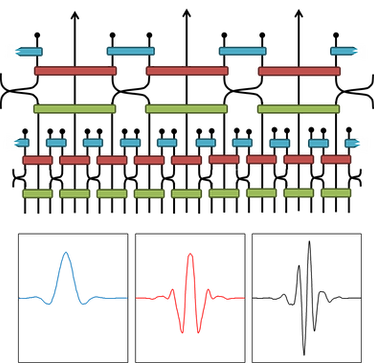top of page## GLEN EVENBLY

My research is focused on the development of novel theoretical and numerical tools for modelling quantum many-body systems.

Research Interests:

• Tensor network states and algorithms

• Real-space renormalization group

• Numerical methods for systems of strongly correlated electrons

• Entanglement and quantum information

• Quantum criticality and phase transitions

• Machine learning and tensor networks

My research website on tensor networks (containing tutorials, example codes and links to further resources) can be found at:

I have designed the TensorTrace software for creating tensor networks and tensor network code, which can be found at:

I am also a developer of the Google TensorNetwork library:

## SOME RECENT RESEARCH PROJECTSTensor Network Classifiers for Superivsed Learning

Many similarities exist between the neural networks used in machine learning and the tensor networks used in the simulation of quantum many-body systems. In this work I propose a restricted class of tensor network state, built from number-state preserving tensors, for supervised learning tasks. This proposal is demonstrated using a variety of benchmark classification problems, where number-state preserving versions of commonly used networks (including MPS, TTN and MERA) are trained as effective classifiers.Hyper-invariant Tensor Networks and Holography:

Tensor network states such as MERA are currently of significant interest as models for holography and, in particular, for the AdS/CFT correspondence. In this work I propose a new class of tensor network state that retain key features of the MERA, including algebraic correlations and efficient contractibility, yet are also built according to a uniform tiling of hyperbolic space, and thus capture the desired symmetries of AdS space.Entanglement Renormalization and Wavelets:

A key result of this project was the establishment of a precise connection between MERA quantum circuits and discrete wavelet transforms (DWTs). This connection gave exciting new insights both into MERA (allowing the first analytic construction of a MERA for the ground state of a critical system) and also into DWTs (allowing construction of improved families of wavelets, with application to more efficient data/image compression).

TensorTrace: a GUI for tensor networks:

TensorTrace is a custom developed, freely available program that I designed to facilitate the development and the implementation of tensor network algorithms. Its main functionality is to provide an easy-to-use graphic drawing interface tailored for the construction of tensor network diagrams. From any completed diagram TensorTrace can automatically generate the code for the optimal contraction of the network (in the users choice of the MATLAB, Python or Julia languages).Implicitly Disentangled Renormalization:

Many powerful methods for the efficient simulation of quantum many-body systems are based on the renormalization group (RG). In this work I propose a new implementation of real-space RG transformations for quantum states on the lattice. This new approach reproduces the key features of entanglement renormalization (ER), but without the need for unitary disentanglers, which allows a variety of numeric simulation algorithms to be substantially improved.Tensor Network Renormalization:

This project involved the development of a new tensor network algorithm for the simulation of quantum many-body systems, dubbed tensor network renormalization (TNR). A significant result was the demonstration that TNR realizes both an accurate and computationally sustainable coarse-graining transformation even at a critical point, whereas previous approaches diverge near criticality. TNR also produces, for the first time, a scale-invariant fixed point for critical systems.

bottom of page